#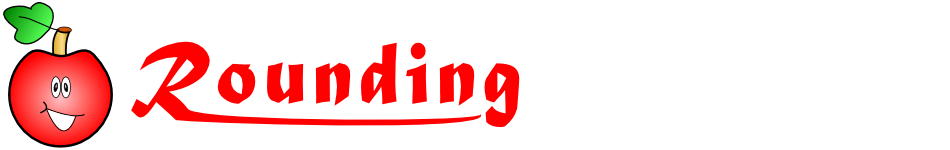There are 366 different Starters of The Day, many to choose from. You will find below some starters on the topic of Rounding. A lesson starter does not have to be on the same topic as the main part of the lesson or the topic of the previous lesson. It is often very useful to revise or explore other concepts by using a starter based on a totally different area of Mathematics.

Main Page

### Rounding Starters: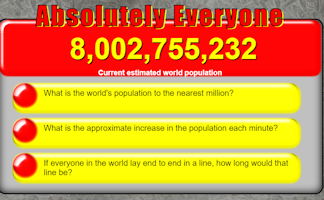A choice of questions about the current world population which is constantly changing.Estimate the distances shown on this photograph of the moon's surface.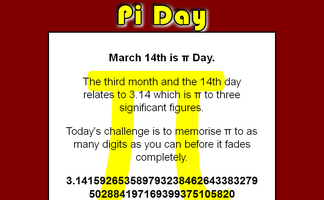March 14th is Pi Day. The third month and the 14th day relates to 3.14 which is pi to three significant figures. How many figures of pi can you memorise?Round off the given numbers to 1 decimal place then add the answers together.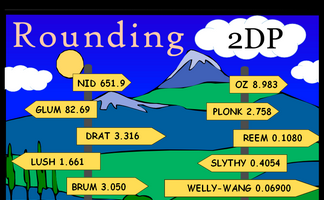Round off the given numbers to 2 decimal places then add the answers together.Round off the given numbers to the nearest whole number then add the answers together.

## Exercises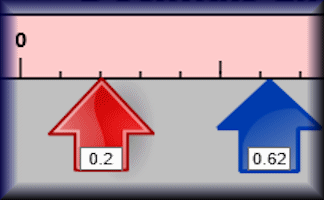#### Decimals Line

A number line showing tenths and hundredths with draggable arrows. This is a visual aid designed to be projected onto a whiteboard for whole class expositionAn exercise on rounding values in a calculation to find an approximate estimate of the answer.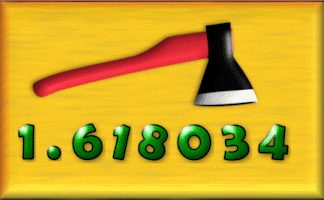#### Rounding DP

A self marking exercise requiring students to round numbers to a given number of decimal places.#### Rounding SF

A self marking exercise requiring students to round numbers to a given number of significant figures.#### Rounding Snap

If the last card put down equals the previous card to the nearest whole number then all players race to shout SNAP!#### Rounding Ten

Round the numbers to the nearest whole number or the given power of ten.#### Upper and Lower Bounds

Determine the upper and lower bounds when rounding or truncating quantities used in calculations.

### Search

The activity you are looking for may have been classified in a different way from the way you were expecting. You can search the whole of Transum Maths by using the box below.

Have today's Starter of the Day as your default homepage. Copy the URL below then select
Tools > Internet Options (Internet Explorer) then paste the URL into the homepage field.

Set as your homepage (if you are using Internet Explorer)

Do you have any comments? It is always useful to receive feedback and helps make this free resource even more useful for those learning Mathematics anywhere in the world. Click here to enter your comments.For All: